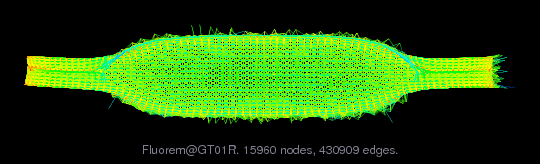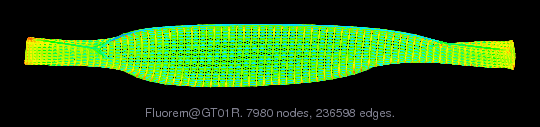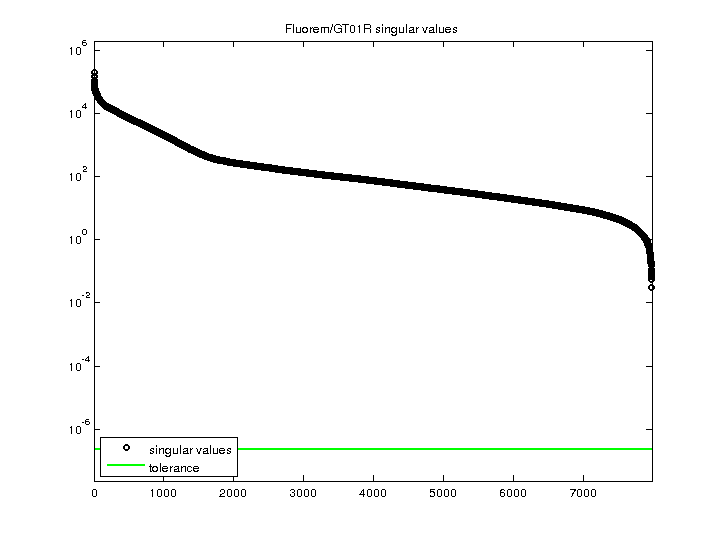Matrix: Fluorem/GT01R

Description: GT01R: 2D inviscid case. F. Pacull, Lyon, France(bipartite graph drawing) (graph drawing of A+A')• Home page of the UF Sparse Matrix Collection
• Matrix group: Fluorem
• Click here for a description of the Fluorem group.
• Click here for a list of all matrices
• Click here for a list of all matrix groups
• download as a MATLAB mat-file, file size: 3 MB. Use UFget(2335) or UFget('Fluorem/GT01R') in MATLAB.
• download in Matrix Market format, file size: 5 MB.
• download in Rutherford/Boeing format, file size: 4 MB.

 Matrix properties number of rows 7,980 number of columns 7,980 nonzeros 430,909 structural full rank? yes structural rank 7,980 # of blocks from dmperm 4 # strongly connected comp. 4 explicit zero entries 0 nonzero pattern symmetry 88% numeric value symmetry 0% type real structure unsymmetric Cholesky candidate? no positive definite? no

 author F. Pacull editor T. Davis date 2010 kind computational fluid dynamics problem 2D/3D problem? yes

 Additional fields size and type b full 7980-by-1 x full 7980-by-1

Notes:

CFD matrices from Francois Pacull, FLUOREM, in Lyon, France

We are dealing with CFD and more precisely steady flow
parametrization. The equations involved are the compressible
Navier-Stokes ones (RANS).  These matrices are real, square and
indefinite, they correspond to the Jacobian with respect the
conservative fluid variables of the discretized governing
equations (finite-volume discretization). Thus they have a
block structure (corresponding to the mesh nodes: the block
size is the number of variables per mesh node), they are not
symmetric (however, their blockwise structure has a high level
of symmetry) and they often show some kind of hyperbolic
behavior. They have not been scaled or reordered.

They are generated through automatic differentiation of the
flow solver around a steady state. A right hand-side is also
given for each matrix: this represents the derivative of the
equations with respect to a parameter (of operation or shape).
Since they are generated automatically, they may have "silent"
variables: these are variables corresponding to an identity
submatrix associated with a null right hand-side, for example
one of the three velocity components in a 2D case, or the
turbulent variables in a "frozen" turbulence case.

We believe that these matrices are good test cases when
studying preconditioning methods for iterative methods, such as
block incomplete factorization, or when studying domain
decomposition methods or deflation. They are actually being
studied by a few researchers in France regarding numerical
methods, through the LIBRAERO research project of the ANR (national
research agency): ANR-07-TLOG-011.

Francois Pacull, Lyon, France.  fpacull at fluorem.com

Specific problem descriptions:
GT01R: 2D inviscid case
number of mesh nodes: 1596
block size: 5
variables: [rho,rho*u,rho*v,rho*w,rho*E]
(rho w is "silent", the fourth row and column in each
block can be removed)
matrix order: 7980
nnz: 430909
comments: This is a 2D linear cascade turbine case. The grid
corresponds to one inter-blade channel. The stencil involved
by the convective scheme uses 9 nodes. Thus, there are 9
non-zero blocks for each node in the matrix. The specificity
is that the computational domain is periodic, which introduces
some non-zeros elements far away from the diagonal.

 Ordering statistics: result nnz(chol(P*(A+A'+s*I)*P')) with AMD 1,446,641 Cholesky flop count 3.3e+08 nnz(L+U), no partial pivoting, with AMD 2,885,302 nnz(V) for QR, upper bound nnz(L) for LU, with COLAMD 2,765,274 nnz(R) for QR, upper bound nnz(U) for LU, with COLAMD 5,518,657

 SVD-based statistics: norm(A) 195007 min(svd(A)) 0.0308668 cond(A) 6.31767e+06 rank(A) 7,980 sprank(A)-rank(A) 0 null space dimension 0 full numerical rank? yes

 singular values (MAT file): click here SVD method used: s = svd (full (A)) ; status: okFor a description of the statistics displayed above, click here.

Maintained by Tim Davis, last updated 12-Mar-2014.
Matrix pictures by cspy, a MATLAB function in the CSparse package.
Matrix graphs by Yifan Hu, AT&T Labs Visualization Group.